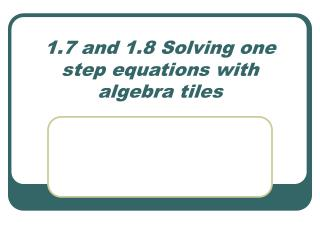DownloadDownload Presentation1.7 and 1.8 Solving one step equations with algebra tiles

# 1.7 and 1.8 Solving one step equations with algebra tiles

Download Presentation## 1.7 and 1.8 Solving one step equations with algebra tiles

- - - - - - - - - - - - - - - - - - - - - - - - - - - E N D - - - - - - - - - - - - - - - - - - - - - - - - - - -
##### Presentation Transcript

1. 1.7 and 1.8 Solving one step equations with algebra tiles

2. Algebra Tiles: 1 • We have 2 types of tiles we will be working with this year: x 1 1 x 1 So, we name the tiles by their areas: x’s and ones (unit tiles).

3. How can we show zero with the tiles? • A zero is a positive and negative tile of the same type. • We show a negative with the tiles by it’s color. The RED side is negative. If we are drawing a picture, the NON-shaded tile is Negative. • Ex: = 0 = 0

4. Solving Equations How to solve equations: *Get the variable (x) by itself. **Legal moves: Why do we need to solve equations? We need to find the value of the variable that makes the equation true. • Make zeros to get rid of unit tiles…put the same # of tiles on BOTH sides of =‘s. • Divvy up unit tiles on one side so EACH “x” tile receives the same # of unit tiles. • If “x” tiles are negative, flip all tiles (once “x” tiles are alone) to their opposite sign. • If an “x” tile is being divided into parts, EACH part has the SAME # of unit tiles in it. Count how many unit tiles are in the WHOLE “x” tile.

5. Examples: a) x + 1 = 3 b) 2x = 6 c) 8 = -4k d) zeros x = 3 So, x = 2 Since a –k = 2, FLIP! k = -2 So, the WHOLE m = -12